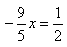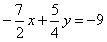# Test: Formation Of Linear Equations

## 20 Questions MCQ Test Mathematics (Maths) Class 9 | Test: Formation Of Linear Equations

Description
This mock test of Test: Formation Of Linear Equations for Class 9 helps you for every Class 9 entrance exam. This contains 20 Multiple Choice Questions for Class 9 Test: Formation Of Linear Equations (mcq) to study with solutions a complete question bank. The solved questions answers in this Test: Formation Of Linear Equations quiz give you a good mix of easy questions and tough questions. Class 9 students definitely take this Test: Formation Of Linear Equations exercise for a better result in the exam. You can find other Test: Formation Of Linear Equations extra questions, long questions & short questions for Class 9 on EduRev as well by searching above.
QUESTION: 1

Solution:
QUESTION: 2

Solution:
QUESTION: 3

### A linear equation in one variable is an equation of the form ………..

Solution:

A linear equation is an equation of a straight line, written in one variable. The only power of the variable is 1. Linear equations in one variable may take the form a x + b = 0, ax+b=0, ax+b=0 and are solved using basic algebraic operations

QUESTION: 4

The Equation y = – 5, in two variables can be written in the form of ax + by + c = 0 as​

Solution:
QUESTION: 5

Which of the following equations is linear equation in two variables?

Solution:
QUESTION: 6

The number of girls x in a class is 5 less than twice the number of boys y of the same class. The linear equation in two variables for this information is

Solution:

Explanation : Let number of girls in class = x

Boys = 2y - 5

Equation will be : x + 5 = 2y

QUESTION: 7

The speed x of train A is twice the speed y of the train B. Express this in the form of a linear equation.

Solution:
QUESTION: 8

The cost of a pencil is thrice the cost of rubber.The equivalent linear equation in two variables of above statement is:

Solution:
QUESTION: 9

The linear equation x = 5 can be written in two variables as:​

Solution:
QUESTION: 10

The equationin two variables is expressed as:

Solution:
QUESTION: 11

Which of the following is linear equation?

Solution:
QUESTION: 12

Which of the following is a linear inequality with two variables?

Solution:
QUESTION: 13

Which of the following is not true?

Solution:
QUESTION: 14

The coefficients a,b, c in the following linear equation of the form ax + by + c = 0 is of the equationare

Solution:
QUESTION: 15

If half of the cake is equal to the six pastries then the linear equation in two variables of above statement is expressed as

Solution:
QUESTION: 16

For an equation ax + by + c = 0 to be a linear equation,which one of the following conditions should be satisfied?

Solution:
QUESTION: 17

Which of the following is a linear equation in one variable?​

Solution:
QUESTION: 18

Which of the following is linear equation?

Solution:
QUESTION: 19

Difference between the cost of a carpet(C) and a durries(D) is Rs.200. If the carpet is the costlier one of the two, which of the following represents this information?

Solution:

The difference in costs of two articles is 200 and since the carpet is more costlier than durry. So the equation will be C - D = 200

QUESTION: 20

Four times B’s age exceeds A’s age by twenty years.

Solution: